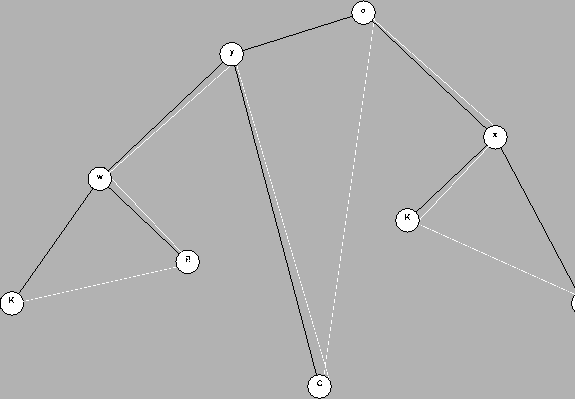Next: How to compute this Up: Probabilistic ancestral sequences and Previous: Probabilistic ancestral sequence.

# Probability of an evolutionary configuration

In this section we will describe how to compute the probability of an evolutionary configuration (EC), that is the probability of a particular alignment of sequences according to a given EPT.

First we should notice that our model of evolution treats each position independently of its neighbours. Hence the probability of an EC will be the product of the probabilities for each of the aligned positions. For each aligned position we have an EPT with one amino acid at each leaf. (We consider the special case of indels later). The tree in Figure 2 represents a slice of the EC at position 4 where sequence 1 has a K, sequence 2 has an R, etc. In this case, the amino acids placed at nodes W, X and Y are the unknown amino acids at the divergence points.Gaston Gonnet
1998-07-14